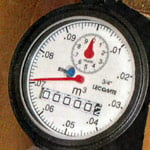###### Taxation# Confirmation of taxes for Professionals

## Valuation Roll and taxes for PROFESSIONNALS(Notaries, financial institutions, real estate agents, appraisers, etc.)

For confirmation of taxes and assessment of a property in Kirkland, please either:

• email us at
• or call 514 694-4100, ext. 3119 during regular business hours
A fee of \$20 is applicable for each tax and assessment confirmation, paid by cheque made out to the Town of Kirkland.

##Water consumption

Please note that in case of sell of a property, a final reading of the water meter is compulsory and must be completed one week prior to the signing date with the notary.
A photo of the water meter must be sent by email to:

## Duties on transfers of immovables

Under provincial legislation, a transfer tax must be collected by the City for the transfer of any immovable located in the City.

## Calculation of transfer taxes

The rate of these duties is set as follows:

Parts of the basis of imposition and corresponding tax rates from January 1, 2018, to July 17, 2018

For the section of the tax base:

• That does not exceed \$50,400, the rate is 0.5%;
• That exceeds \$50,400 but does not exceed \$251,800, the rate is 1%;
• That exceeds \$251,800, the rate is 1.5%.

Calculation example for a tax base of \$1,100,000

1.     Multiply \$50,400 by 0.5 % = \$252
2.     Multiply \$201,400 by 1 % = \$2,014
3.     Multiply \$848,200 by 1.5 %  = \$12,723
4.     So, for a tax base of \$1,100,000, the transfer duties amount to: \$14,989.

Parts of the basis of imposition and corresponding tax rates starting July 18, 2018

For the section of the tax base:

•     That does not exceed \$50,400, the rate is 0.5%;
•     That exceeds \$50,400 but does not exceed \$251,800, the rate is 1%;
•     That exceeds \$251,800 but does not exceed \$500,000, the rate is 1.5%.
•     That exceeds \$500,000 but does not exceed \$1,000,000, the rate is 2%.
•     That exceeds \$1,000,000, the rate is 2.5%.

Calculation example for a tax base of \$1,100,000

1.     Multiply \$50,400 by 0.5 % = \$252
2.     Multiply \$201,400 by 1 % = \$2,014
3.     Multiply \$248,200 by 1.5 %  = \$3,723
4.     Multiply \$500,000 by 2 %  = \$10,000
5.     Then, multiply \$100,000 by 2.5 %  = \$2,500
6.     So, for a tax base of \$1,100,000, the transfer duties amount to: \$18,489

Parts of the basis of imposition and corresponding tax rates starting January 1, 2019

For the section of the tax base:

• That does not exceed \$50,900, the rate is 0.5%;
• That exceeds \$50,900 but does not exceed \$254,400, the rate is 1%;
• That exceeds \$254,400 but does not exceed \$500,000, the rate is 1.5%.
• That exceeds \$500,000 but does not exceed \$1,000,000, the rate is 2%.
• That exceeds \$1,000,000, the rate is 2.5%.

Calculation example for a tax base of \$1,100,000

1. Multiply \$50,900 by 0.5 % = \$254.50
2. Multiply \$203,500 by 1 % = \$2,035
3. Multiply \$245,600 by 1.5 % = \$3,684
4. Multiply \$500,000 by 2 % = \$10,000
5. Then, multiply \$100,000 by 2.5 % = \$2,500
6. So, for a tax base of \$1,100,000, the transfer duties amount to: \$18,473.50

## Payment of transfer taxes

This tax is payable in one installment within thirty (30) days of the mailing of the tax bill by the City. The taxation basis transfer duties will be the greatest of the following amounts:

• The amount of the counterpart provided for the transfer of the immovable
• The amount of the counterpart stipulated for the transfer of the immovable
• The amount of market value at the time of the transfer of the immovable. As mentioned in the Act respecting duties on transfers of immovables, the market value is the product obtained by multiplying the value entered on the roll for the unit or part corresponding to the transferred immovable, as the case may be, by the factor of the roll established.